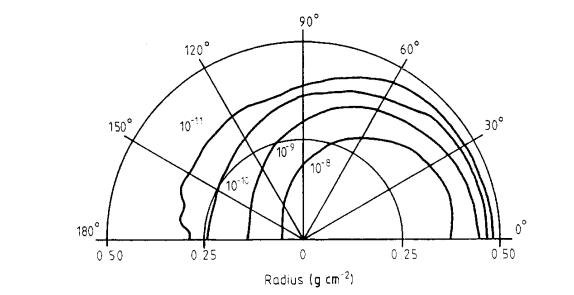# Drawing Polar plot

hi dear experts,

I want to draw something like this :I have a 2D histogram that represents radius and angle.

I drew it by using the POL option but it did not work. I think I may define angle interval and draw counter lines by using the cont3 option. if it is correct I don’t know how can I define angle intervals and draw counter as a function of radius and angle.

how can I do this?

I attach my .root file, there is a 2d histogram in which 0-48 represents 48 angle intervals (3.75 degrees) and 0-24 represents radiuses.

result.root (5.2 KB)

many thanks,
Keyvan

There is no simple option producing such plot (half circle) with contour plots from a TH2…
The Pol option is one way to represent polar plots. Also there is TGraphPolar. But none of them will produce such plot.

1 Like

the picture that I sent, shows a half-circle plot because of its data, dawing half-circle is not critical, It can be a circle with data that are in a half-circle.

what is important is drawing a counter-polar plot.

like this :

Draw(“LEGO2 POL”) gives me:

I am not sure that’s what you are looking for.

1 Like

i drew it by Draw(“LEGO2 POL”) but i got this :

why my result and your result is different?

thank you it is almost what i want, for achieving an accurate plot i must multiple each 0-48 degrees intervals by 3.75 for creating angle intervals and create another 2D histogram with new angle intervals and radius.

how can i represent radiuses and angles?, like the second picture that i sent.

radiuses are represented on cerntral axis.

many thanks,

I changed the viewing angles…you can try interactively. Without POL I get:

1 Like

This topic was automatically closed 14 days after the last reply. New replies are no longer allowed.